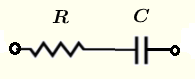# Series RC circuit Impedance Calculator

  

A calculator to calculate the equivalent impedance of a resistor and a capacitor in series. The calculator gives the impedance as a complex number in standard form and polar forms.

## Formulae for Series R C Circuit Impedance Used in the Calculator and their UnitsLet $f$ be the frequency, in Hertz, of the source voltage supplying the circuit.
and define the following parameters used in the calculations
$\omega = 2 \pi f$ , angular frequency in rad/s

$X_C = 1 / (\omega C)$ , the capacitive reactance in ohms $(\Omega)$
The impedance of the capacitor $C$ is given by
$Z_C = \dfrac{1}{j \omega C} = -\dfrac{j}{\omega C}$
Let $Z$ be the equivalent impedance to the series RC circuit shown above and write it in complex form as follows
$Z = R + Z_C$
$Z = R - \dfrac{1}{\omega C} j$
The formulae for the modulus $|Z|$ and argument (or phase) $\theta$ of $Z$ are given by

Modulus: $|Z| = \sqrt{ R^2 + \dfrac{1}{\omega^2 C^2} }$ in ohms $(\Omega)$

Argument (Phase): $\theta = \arctan ( - \dfrac{1}{R \omega C} )$ in radians or degrees

## Use of the calculator

Enter the resistance, the capacitance and the frequency as positive real numbers with the given units then press "calculate".

 Resistance R = 50 mΩ Ω KΩ MΩ Capacitance C = 0.47 pF nF μF mF F Frequency f = 1.5 GHz MHz kH Hz mHz Number of Decimals 4